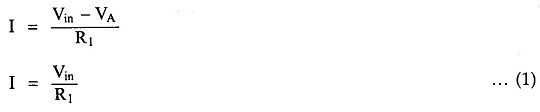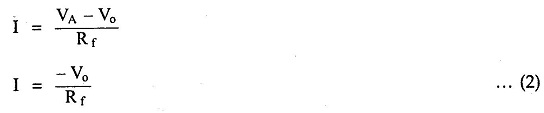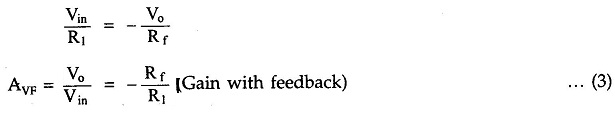# Inverting Amplifier

## Inverting Amplifier Circuit Diagram:

As the name suggests the output of such the input signal. The inverted output signal compared to the input signal. So, an amplifier which provides a phase is called inverting amplifier.

So, an amplifier which provides a phase is called inverting amplifiers Circuit.The basic circuit diagram of an inverting amplifier Circuit using op-amp is shown in the Fig. 2.17 (a).
Let us derive the expression for its closed loop gain which is Vo / Vi„

As node B is grounded, node A is also at ground potential, from the concept of virtual ground, so VA = 0Now from the output side, considering the direction of current I we can write,Entire current I passes through R f as op-amp input current is zero.

Equating (1) and (2) we get,The Rf/R1 is the gain of the amplifier while negative sign indicates that the RI polarity of output is opposite to that of input. Hence it is called inverting amplifier Circuit. The input and output waveforms are shown in the Fig. 2.17 (b).